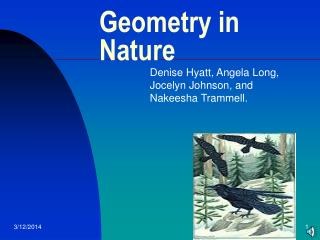DownloadDownload PresentationGeometry in Nature

# Geometry in Nature

Download Presentation## Geometry in Nature

- - - - - - - - - - - - - - - - - - - - - - - - - - - E N D - - - - - - - - - - - - - - - - - - - - - - - - - - -
##### Presentation Transcript

1. Geometry in Nature Denise Hyatt, Angela Long, Jocelyn Johnson, and Nakeesha Trammell.

2. Introduction • Discovering the link between geometry and nature is not a difficult task. • Geometry is everywhere in the natural environment • Geometry in Nature is also known as “Fractal Geometry” developed by Benoit Mandelbrot.

3. Nature’s highest points in Geometry • Fibonacci Numbers and Nature • Solar System • Reflectional symmetry • Weather • The Nazca Lines

4. Fibonacci and Nature • Fibonacci numbers describes a series of numbers in which successive numbers is equal to the sum of the two proceeding numbers. • Examples range from: animals, vegetables, and flowers.

5. Fibonacci and Nature Con’t: • The Echinacea purpura flower, also known as the cone flower. • The orange petals follow Fibonacci’s pattern by the spirals curving left and right. • The numbers in each spiral follows the neighboring pattern of Fibonacci’s sequence.

6. Solar Geometry • The distance from each planet from the sun can be described by a unique geometric formula. • The orbital distance between each planet from the sun is calculated by using various geometric shapes, which include: square sides, radius of a circle, equilateral triangles, and isosceles triangles. • Four examples are: Mecury and Venus, Venus and Earth, Earth and Mars, and Mars and Jupiter.

7. Solar Geometry Con’t: • reflection of light • Even though the discovery of the geometrical basis for all nature’s designs were publised by Fuller more than 30 years ago, todays society still is not aware of this huge achievement. • Energy cannot stand still , it is always in motion, so it is constantly moving in some direction. • Since energy is traveling in a direction for a specific amount of time, it is called a “vector”. • Anything with an inside and outside is called a system, and the minimum system in the universe has to be a tetrahedron.

8. Reflectional Symmetry • Reflectional symmetry is shown in many areas of nature • These include moths, the human body, and fruit.

9. Weather • As snow crystals form, they become a hexagonal shape, it seems like an infinite number of variations for being six-sided. • The temperature that the crystals forms, and to less extent the humidity of the air, determine the basic shape. • Snow crystals form a hexagonal shape because of the way the two hydrogen atoms join with an oxygen atom to form a water molecule.

10. The Nazca Lines

11. The Nazca Line • Discovered in 1930 • Scattered over 500 square km • Contains over 50 “geoglyphs” • Over 1,300 km straight lines • 300 geometric shapes

12. “Geoglyphs” • These geometrical forms include straight lines, triangles, spirals, circles,trapezoid and depiction of animals and plants

13. “Pampas” Desert with little wind covered with black wind-smoothed rock Lines were created by removing the black fragments and topsoil How Are They Formed?

14. The Nazca Lines • The Nazca lines can be as narrow as 6 inches and as wide as hundreds of yards.

15. Thunderbird • One of the most famous geoglyphs • Lines are formed by one continuous line

16. Intersecting Lines • These two Nazca Lines are intersecting because they share a common point.

17. Trapezoid • Wedge-like • This trapezoid has sides that are 2,500 ft long • Its sides run parallel for about 5 miles

18. Theories of the nazca Lines • Space craft landing • Population control systems • Running footraces • Astronomical purposes

19. Fractal Geometry • Developed by Benoit Mandelbrot’s study of complexity and chaos.

20. Modern Uses • Landscaping • Intricate models • Images of natural scenes • Mountain ranges and coast lines